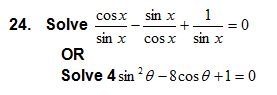Home » Science » Mathematics » Sample Paper of Mathematics 2014 for class 11, CBSE. Paper No.2

# Sample Paper of Mathematics 2014 for class 11, CBSE. Paper No.2

Sample Paper – 2014
Class – XI
Subject – Mathematics

Maximum Time– 3 Hrs                                                                                 Maximum Marks – 100

General instructions –

• All questions are compulsory.

• The question paper consists of 29 questions divided in to three sections A, B and C. Section A comprises of 10 questions of 1 mark each, Section B comprises of 12 questions of 4 marks each and Section C comprises of 7 questions of 6 marks each.

• All questions in Section A are to be answered in one word, one sentence or as per the exact requirement of the question.

• There is no over all choice. However, internal choice has been provided in 4 questions of four marks each and 2 questions of six marks each. You have to attempt only one of the alternatives in all such questions.

• Use of calculators is not permitted

SECTION – A

1. Write the set in the set builder form.
2. A relation R is defined on the set of integer as R =find two elements of R.
3. Given 5 flags of different colours, how many different signals can be generated if each signal requires the use of two flags, one below the other?

1. If P(n) : 6n – 5n – 1  is divisible by 25,  check its validity for n = 3.
2. Find the modulus  of (2-3i)
3. Evaluate cos(-1710° ).
4. Find the solution of the in equation 2x – 5  ≤  3x + 7
5. If the no. terms in the expansion ( a + b )3m – 1 are 20, then find m .
6. Find two numbers whose AM=34 and GM=16.
7. Find the value of K such that the line joining the points (2, K) and (-1, 3) is parallel to the line joining (0, 1) and (-3, 1)

SECTION – B

1. Let f =    be a function from R into R. Determine the range of f.
2. If sinx=find the value of
3. Prove that cos 2x  cos

1. Prove by mathematical induction

1+

1. Convert the complex number into polar form.

OR

Solve       x 2 –  (3

1. If   11 P r    = 12  P  r-1   find r

OR

In how many distinct permutations of the letters in MISSISSIPPI do the four I’s not come together.

1. On the occasion of friendship day 65 cadets purchased F’bands for each other. They tied the bands to each other and shook hands. Find the total number of hand shakes that occurred

1. Find the sum to n terms of the series: 5+11+19+29+41……………………
2. The sum of two number is 6 times their geometric mean, show that numbers are in the ratio
3. Find the coordinates of point on y –axis which are at a distance of 5from the point  P(3,-2,5)
4. Solve the following system of inequalities graphically

1. In If P (A ) = P ( B ),  show that    A  = B

SECTION – C

1. In a survey of 100 persons it was found that 28 read The New Indian Express, 30 read The Statesman, 42 read The Hindu, 8 read TNIE and TS, 10 read TNIE and TH, 5 read TS and TH and 3 read all three.

Find:

1. How many read none of three magazines?
2. How many read magazine C only?1.  IF a , b, c are in AP. Prove that 1/bc, 1/ac,1/ab are also in AP.
1. If the third, fourth and fifth  terms in the binomial expansion (x+a)n are 84,280 and 560 respectively, find x,

a and n.

1. Find the image of the point (3,8) with respect to the line x+3y=7 assuming the line to be a plane mirror.

1. A manufacturer has 600 litres of a 12% solution of acid. How many litres of a 30% acid solution must be  added to it so that acid content in the resulting mixture will be more than 15% but less than 18%?

1. The coefficient of the ( r-1 )th , rth and ( r + 1 )th terms in the expansion of ( x + 1 )n are in the ratio 1 : 3 : 5 . Find n  and  r ?

Paper Submitted by:

Name: PRS Chakravarthi

Email: prschakravarthi@yahoo.co.inThe main objective of this website is to provide quality study material to all students (from 1st to 12th class of any board) irrespective of their background as our motto is “Education for Everyone”. It is also a very good platform for teachers who want to share their valuable knowledge.
1.kamal kant chaturvedi says: